## Saturday, January 13, 2007

### Solving Van Roomen's Problem: Step Three

The third step in solving Van Roomen's problem is realizing its relation to trigonometry. Using Fn(x) defined in my last blog (see here), I will show that:

2cos(nα) = Fn(2cosα) where n ≥ 1.

and

2sin(nα) = (-1)(n-1)/2Fn(2sinα) where n is odd and n ≥ 1.

Here are the details:

Lemma 1: n ≥ 1 → 2 cos(n)α = (2cos α)(2 cos (n-1)α) - 2 cos(n-1)α

Proof:

(1) From the addition and subtraction cosine formulas [see Theorem 1, here], we know that:

cos (a + b) = cos a cos b + sin a sin b

cos (a - b) = cos a cos b - sin a sin b

(2) Adding these two identities together gives us:

cos (a + b) + cos (a - b) = cos a cos b + cos a cos b = 2cos a cos b

Or in other words:

cos (a + b) = 2 cos a cos b - cos (a - b)

(3) Since a,b can be any value, let b = α, let a = (n-1)α

So that:

a + b = (n-1)b + b = (n-1+1)b = nα

a - b = (n-1)b - b = (n-2)α

(4) This then gives us that:

cos (nα) = (cos α)(2 cos(n-1)α) - cos(n-2)α

Or equivalently:

2 cos (nα) = (2 cos α)(2 cos(n-1)α) - 2 cos(n-2)α

QED

This trigonometric identity is relevant to Van Roomen's problem because it fits the same structure as Fn

In my previous blog, I showed that Fn(x) = x*Fn-1(x) - Fn-2(x).

Now, if x = 2 cos α, then we have:

Fn(2 cos α) = (2 cos α)*Fn-1(2 cos α) - Fn-2(2 cos α)

This brings us to the corollary:

Corollary 1.1: 2 cos(nα) = Fn(2 cos α)

Proof:

(1) At n = 1:

F1 = x = 2 cos α = 2 cos(n α)

(2) At n = 2:

F2 = x2 - 2 = (2 cos α)2 - 2 = 2(2 cos2(α) - 1)

Since sin2(x) + cos2(x) = 1 (see Corollary 2, here), we have :

2(2 cos2(α) - 1) = 2(2 cos2(α) - [sin2(α) + cos2(α)] ) = 2[cos2(α) - sin2(α)]

Using the formula for cos(2x) (see Lemma 3, here), we get:

2[cos2(α) - sin2(α)] = 2 (cos 2 α)

(3) So, let's assume that Fn(2 cos α) = 2 cos n α up to n-1 where n ≥ 3.

(4) Now, using our previous formula (see Theorem 1, here), we know that:

Fn(x) = x*Fn-1(x) - Fn-2(x)

(5) Using our assumption in step #3, we have:

Fn(2 cos α) = (2 cos α)*(2 cos(n-1)α) - (2 cos(n-2)α)

(6) Now, using Lemma 1 above, we know that:

2 cos nα = (2 cos α)*(2 cos (n-1)α) - (2 cos(n-2)α) so that by induction (see Theorem, here for review if needed), we have proven that:

Fn(2 cos α) = 2 cos n α

QED

But we are not yet done, we can also show:

Corollary 1.2: For all odd n ≥ 1:

2 sin nα = (-1)(n-1)/2Fn(2 sin α)

Proof:

(1) From a previous result (see Lemma 1, here), we know that:

cos(z) = sin(z + π/2) where z is any number in radians (see here for review of radians).

(2) Let z = x - π/2

(3) Then:

cos(x - π/2) = sin(x - π/2 + π2) = sin(x)

(4) Now from Corollary 1.1 above, we have:

2 cos(nα) = Fn(2 cos α)

(5) Since α can be any value let α = β - π/2

so that:

2 cos(n[β - π/2]) = 2 cos(nβ - nπ/2) = 2 cos([nβ - (n-1)π/2] - π/2) = 2 sin(nβ - (n-1)π/2)

and

Fn(2 cos (β - π/2)) = Fn(2sin(β))

(6) Using the formula for sin(a+b) [see Theorem 1, here], we get:

sin(nβ - (n-1)π/2) = sin(nβ)cos([n-1]π/2) - cos(nβ)sin([n-1]π/2)

(7) Since n is odd, we know that n-1 is even and there exists m such that (n-1)=2m which gives us:

cos([n-1]π/2) = cos(mπ) = (-1)m since:

(a) n ≥ 1 so m ≥ 0.

(b) cos(0) = 1, cos(π)= -1 [See Property 6, here]

(c) Finally cos(x + 2π) = x [See Property 5, here]

(d) If m is even, then is divisible by and cos(mπ) = 1 = (-1)m

(e) If m is odd, then is not divisible by and cos(mπ) = -1 = (-1)m

Likewise:

sin([n-1]π/2) = sin(mπ) = 0 since:

(a) n ≥ 1 so m ≥ 0.

(b) sin(0) = 0, sin(π) = 0 [see Property 1, here]

(c) sin(x + 2π) = sin x [See Property 5, here]

(d) Putting this together, we can see that mπ ≡ 0 or ≡ π (mod 2π) and either way sin(mπ)=0.

(8) So that we have:

2 sin(nβ - (n-1)π/2) = 2*[sin(nβ)cos([n-1]π/2) - cos(nβ)sin([n-1]π/2)] = 2*(-1)(n-1)/2*sin(nβ) - cos(nβ)*0 = 2*(-1)(n-1)/2sin(nβ)

(9) Using step #8 and combining it with step #4 and step #5 gives:

2*(-1)(n-1)/2sin(nβ) = Fn(2sin(β))

(10) Multiplying both sides by (-1)(n-1)/2 gives us:

2sin(nβ) = (-1)(n-1)/2Fn(2sin(β))

QED

References

## Thursday, January 11, 2007

### Solving Van Roomen's Problem: Step Two

In my previous blog, I showed how Van Roomen's problem could be reduced to a summation formula:

(45)x - (3,795)x3 + (95,634)x5 - (1,138,500)x7 + (7,811,375)x9 - (34,512,075)x11 + (105,306,075)x13 - (232,676,280)x15 + (384,942,375)x17 - (488,494,125)x19 + (483,841,800)x21 - (378,658,800)x23 + (236,030,652)x25 - (117,679,100)x27 + (46,955,700)x29 - (14,945,040)x31 + (3,764,565)x33 - (740,259)x35 + (111,150)x37 - (12,300)x39 + (945)x41 - (45)x43 + x45 =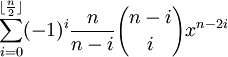where n = 45.

In today's blog, I will show how a recurrence relation can be introduced to simplify the problem further.

The key insight comes to generalizing the above summation formula. For example, let's define:

Fn(x) =It turns out that it is possible to show that for any n ≥ 1:

2cos(nα) = Fn(2cosα)

For any odd n ≥ 1:

2 sin(nα) = (-1)(n-1)/2*Fn(2sinα)

François Viète knew both of these identities and he would use them to find his solutions to Van Roomen's problem. In today's blog, I will show the proof for the recurrent relation and in my next blog, I will show how this recurrence relation leads to the trigonometric identities above.

Theorem 1: Recurrence Formula

If:

Fn(x) =Then:

Fn(x) = x*Fn-1(x) + Fn-2(x)

Proof:

(1) F1(x) = (-1)0*(1/1)*[(1!)/(0!1!)]x1-0 = x

(2) F2(x) = (-1)0*(2/2)*[(2!)/(0!2!)]x2-0 + (-1)1*(2/1)*[(1!)/(1!0!)]x2-2 = x2 - 2

(3) F3(x) = (-1)0*(3/3)*[(3!)/(0!3!)]x3-0 + (-1)1*(3/2)*[(2!)/(1!1!)]x3-2 = x3 - 3x

(4) We can see that x*F2(x) - F1(x) = x*(x2 - 2) - x = x3 - 3x.

(5) So, we can assume that the recurrence formula is true up to some integer n-1 where n ≥ 4, that is:

Fn-1(x) = x*Fn-2(x) - Fn-3(x)

(6) Now, x*Fn-1(x) =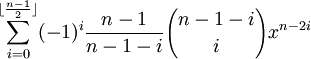=(7) Likewise, Fn-2(x) ===(8) So, we see that: x*Fn-1(x) - Fn-2(x) =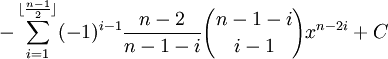where if n is odd,

C = 0

and if n is even,

C =

-(-1)
(n/2 - 1)[(n-2)/(n-1-n/2)][(n-1-n/2)!/(n/2-1)!(n-1-n/2-(n/2-1))!]xn-2(n/2) =

= (-1)(n/2)[(n-2)/(n/2-1)][(n/2-1)!/(n/2 -1)!(0!)]x0 =

= (-1)(n/2)[(n-2)]/[(n/2-1)](1)(1) = (-1)(n/2)(n-2)*(2)/(n-2) =

= (-1)(n/2)*2

(9) So, x*Fn-1(x) - Fn-2(x) - C - xn =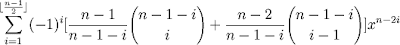(10) Focusing solely on the middle part, we see that:(11) If n is odd:

Fn(x) =(12) If n is even:

Fn(x) =(13) So, either way we have:

Fn(x) = x*Fn-1(x) - Fn-2(x).

QED

### Solving Van Roomen's Problem: Step One

In the last blog, I presented Van Roomen's Problem:

(45)x - (3,795)x3 + (95,634)x5 - (1,138,500)x7 + (7,811,375)x9 - (34,512,075)x11 + (105,306,075)x13 - (232,676,280)x15 + (384,942,375)x17 - (488,494,125)x19 + (483,841,800)x21 - (378,658,800)x23 + (236,030,652)x25 - (117,679,100)x27 + (46,955,700)x29 - (14,945,040)x31 + (3,764,565)x33 - (740,259)x35 + (111,150)x37 - (12,300)x39 + (945)x41 - (45)x43 + x45 = A

where:Each of the coefficients is large and none of them are prime. Nineteen of them, for example, are divisible by 5. 95,634 is divisible by 7. 236,030,652 is divisible by 12. 740,259 is divisible by 3.

In addition, if we examine the coefficients in series from x1 thru x45, we can see that they are smallest at the edges and progressively larger toward the middle. This is a pattern also exhibited by binomials such as (x + y)n.

François Viète was able to reformulate's Adriaan van Roomen's equation into a summation equation. Using modern notation, he reduced the problem to: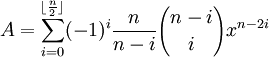where:

n = 45= the largest integer ≤ n/2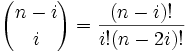[See here for review of factorial (!) if needed]

The floor(n/2) function is used because we only want to generate 22 different terms. Van Roomen's equation consists solely of odd powers.

If you want to see that it works, you can work it out for each term. Here are some examples of how the summation formula works:

At i=0:

(-1)0*(45/45)*[(45)!/(0!45!)]x45 = x45

At i=1:

(-1)1*(45/44)[(44)!/(1!43!)]x43 = -45x43

• Jean-Pierre Tignol, Galois' Theory of Algebraic Equations, World Scientific, 2001